## A CATALOG OF RADIATION HEAT TRANSFER CONFIGURATION FACTORS

John R. Howell
University of Texas at Austin

## INTRODUCTION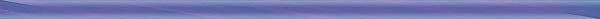Standard texts on general heat transfer and on radiative heat transfer treat the concept of radiation configuration factors in some detail.

The texts discuss various methods of computing these factors and provide examples of the use of configuration factor algebra for generating factors from factors that are already available. However, because of obvious space limitations, only a few of the hundreds of configuration factors that have been derived and published in the engineering literature are reproduced in most of these references.

Many of the early catalogs and references that presented common configuration factors are now out of print or difficult to obtain, and particular factors are scattered throughout the technical literature dealing with basic thermal radiative transfer and the engineering design of lighting systems. In addition, some factors are found in journals concerned with fires and flame spread, solar energy, industrial furnace design, spacecraft thermal control, and others.

This catalog is an attempt to gather many of the most useful published factors into a single source.

Errors identified in earlier publications have been corrected whenever found, and many factors have been rechecked; however, it was not feasible to recompute all previously published factors, particularly results given in graphical form as calculated by numerical integration. The user should therefore be cautious in using the factors, and should point-check factors, particularly those taken from tables and graphs.

In many cases, errors in original references have been reprinted in subsequent compilations or references (see, for example, the discussion in Feingold and Gupta, 1970.) Where these errors are known, only the references with correct values are given in the reference list provided here. Similarly, some factors have been recomputed by subsequent researchers because of the need for improved accuracy or the emergence of better computational or analytical methods. The best data and/or simplest forms in the opinion of the author are selected for presentation here.

The configuration factor is defined as the fraction of diffusely radiated energy leaving surface A that is incident on surface B. No factors are presented for nondiffuse surfaces, although for a certain subset of geometries the factors given here are appropriate for nondiffuse surfaces (particularly certain ones with rotational symmetry).

### NOTATION AND CONFIGURATION FACTORS BETWEEN DIFFERENTIAL ELEMENTS

The notation adopted here is that used in Siegel and Howell. The configuration factor from a differential area element dA1 to a second element dA2 is denoted by dFd1-d2. In general, such a factor is given by

where the quantities on the right-hand-side are shown in Fig. 1.

The differential form dF for element-to-element configuration factors is used to maintain the differential order of the equation to agree with the differential area on the right-hand-side.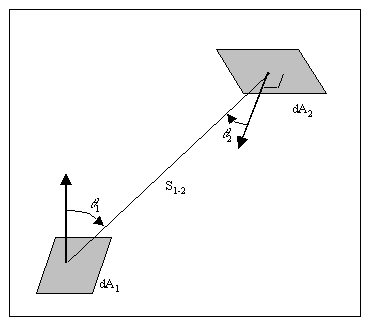FIGURE 1: DEFINING GEOMETRY FOR CONFIGURATION FACTOR.

Eq. (1) can also be put in the form

##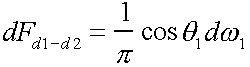(2)

where dw is the solid angle subtended by the projected area dA2 to dA1; that is

##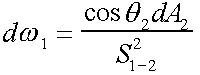(3)

It is easily shown that a reciprocity relation exists:

##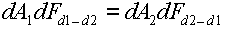(4)

### CONFIGURATION FACTOR FOR A DIFFERENTIAL ELEMENT AND A FINITE AREA

If the receiving area is finite, then the configuration factor from differential surface element dA1 to finite receiving area A2 is given by:

##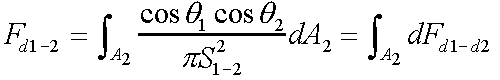(5)

The reciprocity relation is

##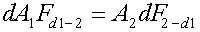(6)

Observe that the choice of notation again keeps the differential order consistent. If the receivingarea is a differential element, then the configuration factor will always be of differential order.

### CONFIGURATION FACTOR FOR FINITE AREA TO FINITE AREA

For the case of A1 and A2 both finite, the configuration factor is

##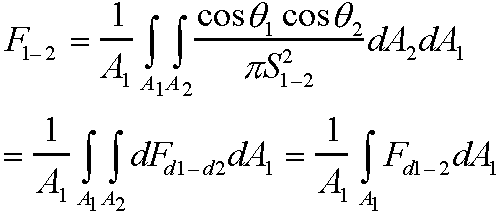(7)

leading to the reciprocity relation

##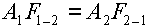(8)

These basic defining equations, Eqs. (1), (5), and (7) are not in the most useful form for a given geometry. It is desirable to have an algebraic relation, or graphical or numerical results that relate the configuration factor to a simple set of parameters that describe the given geometry. Providing such relations is the purpose of this catalog.

### CONFIGURATION FACTOR ALGEBRA

When the configuration factor FA-B between two surfaces is known, the reciprocity relation (Eq. (8)) can be used to find FB-A. Other relations can also be developed that allow simple calculations of new factors from known factors.

If surface B can be subdivided into N nonoverlapping surfaces that completely cover surface B, then

##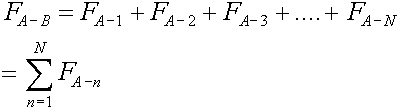(9)

because all energy fractions from surface A to parts of surface B must equal the fraction of the total energy leaving A that is incident on all of B.

Suppose that surface 1 is completely enclosed by a set of M surfaces. In that case, all energy leaving surface A must strike some other surface forming the enclosure. In terms of configuration factors,

##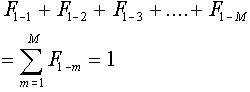(10)

Note the term F1-1 in the summation, which must be included if surface A is concave to account for the fraction of energy leaving surface A that is incident on itself.

The reciprocity relations plus Eqs. (9) and (10) form the basis of what is called configuration factor algebra. Using these relations, new factors can be computed from a small set of known factors; sometimes, factors can be generated from the algebra alone. The procedure is best illustrated by example.

Consider two right isosceles triangles that are joined along their short side as shown in Figure 2. The triangles are perpendicular to one another.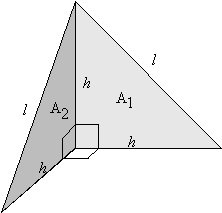FIGURE 2: Perpendicular Right Isosceles Triangles Joined Along Their Short Sides.

To find F1-2, note that an enclosure can be formed by first joining the free corners of the triangles by a line of length l as shown in Figure 3. This forms a corner cavity with the third congruent triangle,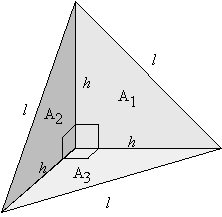FIGURE 3: Construction of Corner Cavity by Addition of Line Connecting Free Corners of Triangle.

The enclosure is completed by placing an equilateral triangle of side l (and area A4) over the cavity formed by the three isosceles triangles, which have equal areas A1, A2, and A3. This is shown in Figure 4.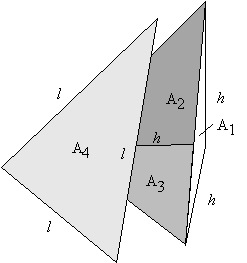FIGURE 4: Completion of Enclosure by Addition of Equilateral Triangle, Surface 4.

Now, apply configuration factor algebra. Eq. 10 gives

##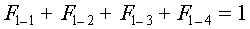(11)

Because surface 1 is planar, F1-1 = 0. By symmetry, F1-2=F1-3. Thus, Eq. (11) reduces to

##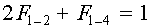(12)

For surface 4 of the enclosure, Eq. (10) plus the use of symmetry gives

##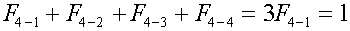(13)

Using reciprocity, Eq. (8), results in

##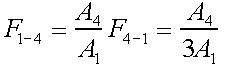(14)

Substituting into Eq. (12) results in

##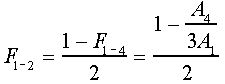(15)

Using geometry, A1=h2/2 and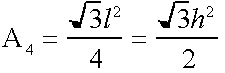, giving

##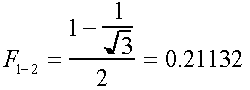(16)

This is the desired answer. The factors 1-4 = 1/(31/2) and F4-1 = 1/3 have also been generated.

Siegel and Howell note that for an N-surfaced enclosure of all planar or convex surfaces (i.e., Fi-i = 0 for all i), N(N-3)/2 factors must be found from a catalog of factors or by calculation. The remaining factors can then be determined by configuration factor algebra. If M of the surfaces (M<N) are concave [i.e., have Fi-i > 0], then [N(N-3)/2]+M factors must be known before configuration factor algebra can determine the remaining factors.

The presence of symmetry may reduce the number of factors that must be known before the rest can be determined.

When the values of certain factors are known approximately, then the constraints imposed on the factors by reciprocity and conservation in an enclosure can be used to refine the known values. Methods for this purpose have been proposed by Sowell and O'Brien, 1972; Larsen and Howell, 1986; van Leersum, 1989; Lawson, 1995; Loehrke et al., 1995; and Taylor et al., 1995.

This catalog is divided into three sections. Section A contains factors between differential elements of the form dFd1-d2; Section B contains factors from a differential area to a finite area, Fd1-2; and Section C provides factors between finite areas, F1-2.

Generally, the catalog is arranged with factors of increasing complexity within each section. Factors within each section begin with planar surfaces to planar surfaces; planar surfaces to cylindrical surfaces; planar surfaces to conical surfaces, spherical surfaces, etc.. Next, factors from cylindrical surfaces to cylindrical surfaces, to conical surfaces, spherical surfaces, etc..

Factors of the form dF2-d1 are not cataloged, but can be obtained by reciprocity from the Fd1-2 factors.

### LIMITATIONS

Many factors have been cataloged here, but others have had to be omitted for various reasons. For example, Grier, 1969, gives about 400 pages of tabular data on configuration factors between spheres and their parts. Abbreviated portions of the data are reproduced here from such compilations, along with a listing of the parameter ranges for which complete data are available in the original reference. The interested reader can thus go to the original source for complete tabulated data.

Many of the early references present extensive tabular or graphical data that were calculated from lengthy closed-form algebraic relations. Present-day computer power and availability makes it easy to compute values, and only the algebraic relations are given in this catalog. Where numerical integration is necessary to obtain results, graphical or tabular data are reproduced in this catalog, although the wide availability of computationally-based engineering programs such as MAPLE, MATHCAD, MATLAB and MATHEMATICA will probably make even these tabulations of little practical use in a few years.Send mail to: John Howell
University of Texas at Austin